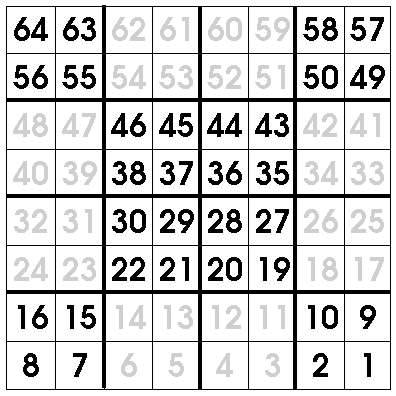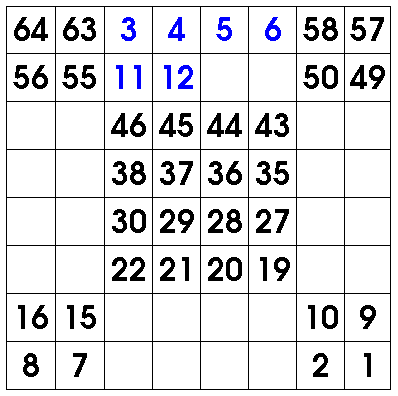# Even Order Magic Square Recipe

### Learn how to build an even order magic square with this simple magic square recipe!

By

Here's a simple magic square recipe that will build magic squares of order four (that is, four by four), or eight (by eight), or twelve - in fact, any order which is a multiple of four. Even 100 by 100! A different page on this site explains how to make a magic square of odd size, and gives some ideas for how you can use the magic squares you find to help teach kids math. Yet another page has a java applet that will help you make a magic square of any size

Step 1 - Prepare Container

Start by drawing a grid of the desired size. Then, divide the grid into 16 equal sized square blocks, as shown below. For a four by four magic square, each block will be a single cell. For a 12 by 12, each block contains nine cells, and so on.Step 2 - Add Flavors

Then, you start writing the numbers 1, 2, 3, and so on into the grid. However, instead of writing from left to right starting from the top, you do the opposite. You also skip certain blocks. That is,

• Starting at the bottom right, write the numbers 1, 2, and so on, moving from right to left. When you reach the end of each row, move to the next row up. However
• You only actually write in the corner blocks and the middle blocks! When you reach cells in the edge blocks, count off the cells, but don't write anything.

If this is a bit confusing, perhaps the picture below will help. The numbers printed in black are the ones actually written. The ones in gray are merely counted off. The last cell you fill should be the top left, and its number should be 16 for a four by four square, 64 for an eight by eight, 144 for a twelve by twelve, 256 for a sixteen by sixteen... that's right - the last number drawn should be the number of cells in the whole grid. (You did just count them, after all.)Step 3 - Blend Well

Then, you fill in the empty squares, this time starting from the top left, and numbering from left to right in the normal way. If a cell already has a number, just count it off. If it's empty, write the number in the cell.Step 4 - Serve Chilled

Finally, you have your magic square!Like the magic squares produced by the other recipe, opposite numbers always add up to some fixed number. Whatever size grid you use,

• Every row, column and diagonal will add up to the same number, called the "magic number" of the square.
• Every pair of opposite cells will add up to the same number. I don't know what this number should be called, so I'll call it the "opposite number". See the picture below, to see what I mean.

I give below the values you should get for the "magic number" and the "opposite number" for different sized squares.

• For a 4 by 4 magic square, the magic number is 34, and the opposite number is 17,
• For an 8 by 8 magic square, the magic number is 260, and the opposite number is 65,
• For a 12 by 12 magic square, the magic number is 870, and the opposite number is 145,
• For a 16 by 16 magic square, the magic number is 2056, and the opposite number is 257,
• For a 20 by 20 magic square, the magic number is 4010, and the opposite number is 401,
• For a 24 by 24 magic square, the magic number is 6924, and the opposite number is 577,
• For a 100 by 100 magic square, the magic number is 500050, and the opposite number is 10001, and
• For a 1000 by 1000 magic square, the magic number is 50000050, and the opposite number is 1000001.

Well, that's all for this even order magic square recipe. I hope it was clear and helpful, and I hope you find good uses for it!

Yours, Dr Mike.

If you like what you've just read, sign up for this site's free newsletters:
• Monday Morning Math: A weekly email of fascinating math facts - how math works in everyday life.
• New Game Alerts: Be first to know when I upload new games.
Get these free now! Enter Your Email: